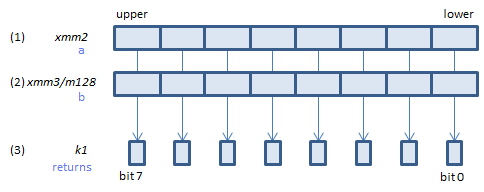﻿ vptestnmw

## VPTESTNMW - Packed TEST Nand Mask Word

VPTESTNMW k1{k2}, xmm2, xmm3/m128    (V5+BW+VLFor each WORD calculate bitwise (1) & (2), if all the bits of the result are 0, set 1, else set 0, to the corresponding bit of (3).
If k2 bit is 0, the calculation is not done and the corresponding bit of (3) is set to zero. Upper bits of (3) are zero cleared.
VPTESTNMW k1{k2}, ymm2, ymm3/m256    (V5+BW+VL